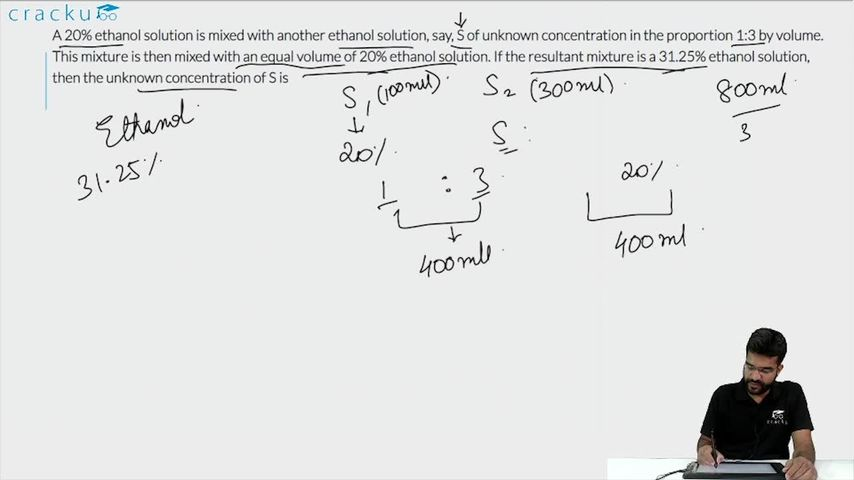Question 75

# A 20% ethanol solution is mixed with another ethanol solution, say, S of unknown concentration in the proportion 1:3 by volume. This mixture is then mixed with an equal volume of 20% ethanol solution. If the resultant mixture is a 31.25% ethanol solution, then the unknown concentration of S is

Solution

Let the volume of the first and the second solution be 100 and 300.
When they are mixed, quantity of ethanol in the mixture
= (20 + 300S)
Let this solution be mixed with equal volume i.e. 400 of third solution in which the strength of ethanol is 20%.
So, the quantity of ethanol in the final solution
= (20 + 300S + 80) = (300S + 100)
It is given that, 31.25% of 800 = (300S + 100)
or, 300S + 100 = 250
or S = $$\frac{1}{2}$$ = 50%
Hence, 50 is the correct answer.

### View Video Solution• All Quant CAT Formulas and shortcuts PDF
• 30+ CAT previous papers with solutions PDF